index: click on a letter A B C D E F G H I J K L M N O P Q R S T U V W X Y Z A to Z index index: subject areas numbers & symbols sets, logic, proofs geometry algebra trigonometry advanced algebra & pre-calculus calculus advanced topics probability & statistics real world applications multimedia entrieswww.mathwords.com about mathwords website feedback

 Integral Rules For the following, a, b, c, and C are constants; for definite integrals, these represent real number constants. The rules only apply when the integrals exist.   Indefinite integrals (These rules all apply to definite integrals as well) 1.2.3.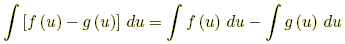4.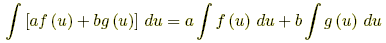5. Integration by parts:1.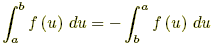2.3. If f(u) ≤ g(u) for all a ≤ u ≤ b, then4. If f(u) ≤ M for all a ≤ u ≤ b, then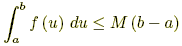5. If m ≤ f(u) for all a ≤ u ≤ b, then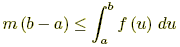6. If a ≤ b, then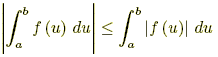See also